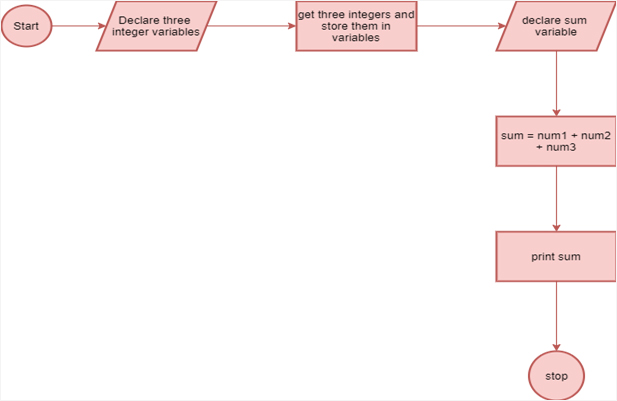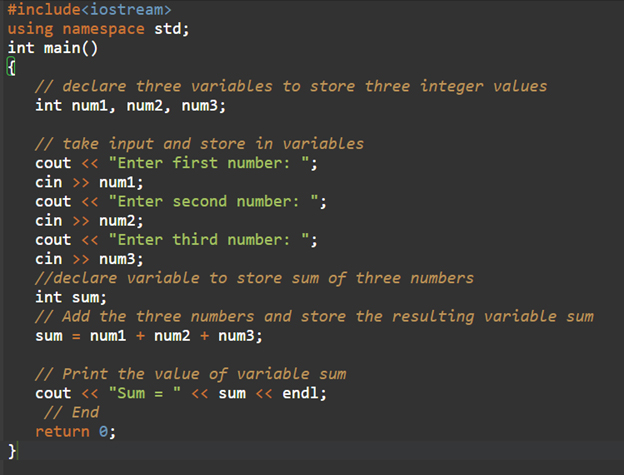# How Does Algorithm Help In Programming?An algorithm is a plan or logical step-by-step process which is used to solve the problem. The algorithms are generally written as a flow chart or in the form of pseudocode.

Docking for solving any problem is to guide the thought process. So, the most helpful thing which we need to do is to keep asking ourselves, what if we did a task in this particular manner.

We can explore different approaches to solve the problem and try to find the best method for solving it. When designing the algorithm, there are two major areas that we can look at:

• The bigger picture which states the final goal
• The individual stages and all the hurdles which need to be overcome to achieve the goal.

## Understanding The Problem

Before starting with the design of the algorithm, we need to check that the problem is understood completely. There are a few basic things which we need to know to understand the problem.

• What will be the inputs in the problem?
• What will be the outputs of the problem?
• The order of the instructions
• What decisions need to be taken in the problem
• Are there any areas of concern that are repeated?

If we are able to understand these basic things, then we can start with the designing of the algorithm.

## Why are Algorithms Important to Understand?

Algorithmic thinking or defining clear steps for solving a problem is crucial in multiple fields. We’re not conscious about it, but we use algorithmic thinking and algorithms in our day-to-day lives too.

The algorithmic thinking will allow the students to break down the problem and conceptualize solutions with the help of discrete steps. First, we need to understand and implement the algorithm, and this can be done if we practice structure thinking and reasoning abilities.

An algorithm can be defined as the step-by-step procedure or the set of rules which we need to follow to complete the specific task or solve a particular problem.

The algorithms are all around us. For example, if you talk about the recipe to bake a cake, solving a long division problem, and the process of doing laundry, these are all few real-life examples of an algorithm.

Here’s are the instructions for making the tea and it is just like an algorithm.

1. Light the stove
2. Keep the pan on it
3. Pour water in it
4. Add tea leaves and sugar accordingly
5. Grate ginger and add it.
6. After a minute, add milk
7. Boil it for 2-3 mins.
8. Take the pan off
9. Get it in cups
10. Enjoy

Algorithmic programming is all about writing a set of rules that can instruct computers on how to perform a task. The computer program is an algorithm that will tell the computer the steps that need to be executed, the specific order of execution, and the task to be performed.

The algorithms are written using a particular syntax based on the programming language which we are using.

We can try to understand it with the help of an example of cooking a new recipe. To cook the new recipe, we need to read the instructions and the steps.

We can execute these steps one by one in the proper given sequence. The result is the new dish cooked to perfection. Similarly, algorithms can help programmers perform the task in programming and get the expected output.

## Features of Algorithm

The algorithms are a set of instructions, and they are language independent. These are the plain instructions that can be further implemented in any language, expecting the same output.

One will not follow any written instructions to perform the task but only the standard ones. Similarly, all the written instructions for the programming cannot be called the algorithm.

In order for some instructions to be considered as the algorithm, it should have the following characteristics.

• Clear and Unambiguous – The algorithm should be unambiguous. Every step should be returning clear aspects and it should result in a single meaningful.
• Well-defined inputs – If the algorithm is taking input, then it should be well-defined inputs.
• Well-defined outputs – The algorithm should be able to define what output can be yielded clearly.
• Feasible – It should be simple, practical, and generic to be executed with the help of available resources. It should not contain any future technology.
• Finite-ness – The algorithm should have finite length and it should not end up in infinite loops.
• Language-Independent – Their designed algorithm should be language-independent, which means that it should be just plain instructions that we can implement in any language and the output will be seen.

## Example

We can consider the following example for adding three numbers and printing the sum.

Step 1

As we have already discussed, the prerequisites must be fulfilled to write an algorithm.

1. The problem which needs to be solved by this algorithm: Add three numbers and print the sum.
2. The constraint of the problem needs to be considered when we’re solving the problem: The numbers should only be digits and no characters are to be used.
3. The input switch needs to be taken for solving the problem: The three numbers to be added.
4. The output to be expected when the problem is solved: The sum of the three numbers.
5. The solution to this problem in the given constraint: We’ll have the addition of three numbers in the solution. We can perform this with the help of the + operator or any other method like bitwise.

Step 2

The second step is to design the algorithm with the help of the above-defined prerequisites.

1. Start
2. Declare three different integer variables like num1, num2 and num3.
3. Get three integers that need to be added as the input in variables num1, num2, and num3.
4. Declare the integer sum for storing the resultant sum of the three numbers.
5. Add the three numbers and store the resulting variable sum.
6. Print the value of the variable sum.
7. End

Step 3

For the testing of this algorithm, we can either implement it using any language or we can do it on pen and paper.

## Flowchart#### C++ Code:#### Output: